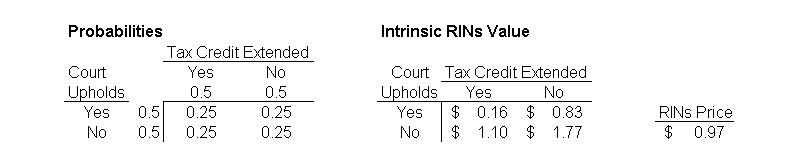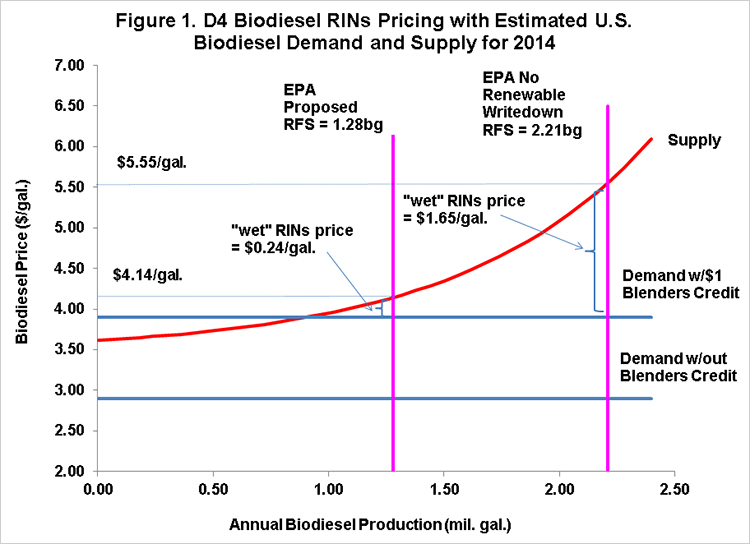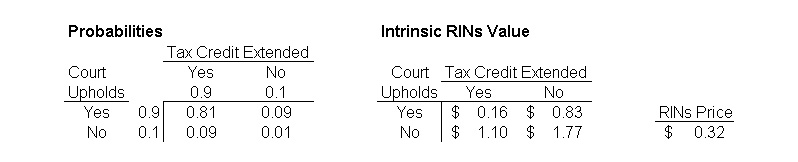# More on Biodiesel RINs Pricing

• Scott Irwin
• Department of Agricultural and Consumer Economics
• University of Illinois
October 30, 2013
farmdoc daily (3):207
Recommended citation format: Irwin, S. "More on Biodiesel RINs Pricing." farmdoc daily (3):207, Department of Agricultural and Consumer Economics, University of Illinois at Urbana-Champaign, October 30, 2013.

A farmdoc daily post last week explored biodiesel RINs pricing in detail. That post showed that biodiesel RINs prices can be thought of conceptually as consisting of two components–intrinsic and time value. The intrinsic value should track current biodiesel blending margins, and it was shown that this is generally true for D4 biodiesel RINS. The time value of RINs reflects the chance that biodiesel blending margins will be even larger (bigger losses) in the future and it has typically in the past represented about one-third of D4 RINs prices. It was also observed that time values had been driven basically to zero in recent weeks, which seems odd given uncertainty about the outcome of EPA rulemaking for 2014 and the status of the blenders tax credit in 2014. The purpose of today’s post is to analyze current D4 biodiesel RINs pricing more formally in light of this uncertainty.

As noted above the two main sources of uncertainty are the outcome of EPA rulemaking for 2014 and the status of the blenders tax credit. The recently leaked EPA proposed rules for 2014 set the biodiesel mandate at 1.28 billion gallons, the same as 2013. Just as important, the proposed 2014 rules write down the renewable (ethanol) mandate from 14.4 billion gallons to 13 billion gallons. Under a binding E10 blendwall of 13 billion gallons and assuming minimal additional E85 deployment in the short-run, the gap between the statutory mandate of 14.4 and the 13 billion gallon blendwall would have, in the absence of a write down, effectively become an additional biodiesel mandate (see the analysis here). Last week’s post used an estimated supply/demand model for biodiesel to show that there is a large difference in D4 RINs prices if the 2014 effective biodiesel mandate is 1.28 or 2.21 billion gallons [1.28 + (14.4 – 13)/1.5)]. The post also showed a large impact on RINs prices if the blenders tax credit is or is not in place for 2014.

Recent media accounts indicate that if the EPA goes ahead with the proposal to write down the renewable mandate in 2014 that the rulemaking will be challenged in court by biofuels groups. This means that biodiesel RINs traders will likely have to assess the probability that a court will uphold the renewable write down for 2014. In addition, traders will have to assess the probability that the blenders tax credit, which will expire at the end of 2013, will be extended for 2014. Assuming the leaked EPA rules are the final proposed rules and the rules are challenged in court, this situation can be formally evaluated using a simple 2 x 2 joint probability table, as shown below:The rows in the left panel represent the two possible outcomes of a court ruling on the 2014 EPA rules, yes they are upheld or no they are struck down. The columns represent the two possible outcomes for extension of the blenders tax credit to 2014, yes it is extended and no it is not extended. As a starting point for the analysis, equal probabilities are assumed in terms of yes/no for the court decision and extending the tax credit. The four innermost cells in the left panel represent the four possible joint outcomes. For example, the upper-left cell represents the outcome of a court upholding the 2014 rules and an extension of the tax credit for 2014. Since the court and tax credit decisions can reasonably be assumed to be independent events, it is easy to compute the joint probabilities for the four combinations of outcomes–simply cross-multiply the relevant yes/no probabilities for each cell. Since the yes/no probabilities are all assumed in this example to be 0.5, the joint probabilities are all 0.25 (0.5 x 0.5). This is a common assumption for probabilities if one assumes “diffuse” knowledge about the likely outcomes. Also notice that the four joint probabilities sum to one.

The next step in the analysis is to determine the intrinsic RINs value for each of the four joint outcomes. These values can be obtained using the supply/demand model presented in last week’s post. Figure 1 presents this model assuming a wholesale diesel price of \$2.90 per gallon and a soybean oil price of \$0.41 per pound, about equal to recent values. The left vertical line on the chart represents the EPA proposed biodiesel mandate of 1.28 billion gallons, which would be in place if a court upholds the write down of the renewable mandate. Under the assumption that the tax credit is extended, the model predicts an equilibrium biodiesel price of \$4.14 per gallon and a wet RINs price of \$0.24 per gallon (\$4.14 – \$2.90 + \$1), which converts to \$0.16 in ethanol equivalents because a “wet” gallon of biodiesel produces 1.5 RINs. If the tax credit is not extended, the wet (ethanol equivalent) RINs price would be \$1.24 (\$0.83). The right vertical line on the chart represents the effective biodiesel mandate of 2.21 billion gallons, which would be in place if a court overturns the write down of the renewable mandate. Under the assumption that the tax credit is extended, the model predicts an equilibrium biodiesel price of \$5.55 per gallon and a wet RINs price of \$1.65 per gallon (\$5.55 – \$2.90 + \$1), which converts to \$1.10 in ethanol equivalents. If the tax credit is not extended, the wet (ethanol equivalent) RINs price would be \$2.65 (\$1.77).We now have all the information needed to compute the “expected” value of a D4 biodiesel RINs given the assumptions up to this point. The right panel of the above table places the RINs values from the supply/demand model in Figure 1 into the appropriate joint “payoff” cells. All that is left to do is to multiply the joint probabilities by the payoffs to determine the “actuarially fair” price for a RINs today:

• \$0.97 = (0.25 x \$0.16) + (0.25 x \$0.83) + (0.25 x \$1.10) + (0.25 x \$1.77).

This set of assumptions predicts a D4 RINs price of \$0.97 per gallon (ethanol equivalents). The high price reflects the influence of combinations of potential outcomes with very high RINs intrinsic values and non-trivial probabilities. This price can be compared to D4 RINs prices actually traded in the market in recent days, in the range of \$0.31 to \$0.33 per gallon. The calculated price is about three times the prices that have been trading in the market. The divergence does not mean one should conclude that market prices for D4 RINs are wrong. Instead, it may simply be the case that traders weight the possible outcomes by different probabilities. The table below presents the same joint probability analysis using yes/no probabilities that result in a computed RINs price that approximately equals recent market prices:All of the assumptions are the same as before except that the probability of yes is assumed to be 0.9 and the probability of a no 0.1 for the court decision and extending the tax credit. The “actuarially fair” price for RINs today under these assumptions is:

• \$0.32 = (0.81 x \$0.16) + (0.09 x \$0.83) + (0.09 x \$1.10) + (0.01 x \$1.77).

This shows that there is a set of probability assumptions that can rationally generate similar D4 prices to that current traded in the market. What the analysis also does is put the focus on most important source of uncertainty in valuing D4 RINs today–the probabilities of the outcomes. While one can quibble to a certain degree with the intrinsic RINs values predicted by the supply/demand model, there should be little argument that RINs values should be relatively high other than in the scenario where the court upholds the 2014 rules and the tax credit is extended for 2014. The key question is how to weight the likelihood of the different outcomes.

#### Implications

The price of D4 RINs has dropped precipitously in recent weeks and now hovers in the low 30 cent range. The decline has driven the time value of the RINs “option” down to essentially zero. The joint probability analysis here shows this is equivalent to assuming there is about a 90 percent chance that a future court ruling will uphold the EPA’s proposed write down of the renewable mandate for 2014 AND about a 90 percent chance that the blenders tax credit will be extended for 2014 by the U.S. Congress and the President. It is hard to know what probabilities to assign to these outcomes, but something near certainty seems optimistic.

November 1, 2013 NOTE: This post has been modified from the original version published on October 30, 2013. There were two small errors in the joint probability tables. The entries for the lower-left and upper-right cells were switched, which does not affect the computed RINs prices. The lower-right cell was incorrectly reported as \$1.70 when the correct value is \$1.77. This increases the first computed RINs price to \$0.97 from the previously reported \$0.92, but does not change the second computed RINs price of \$0.32. Overall, the errors only have a minor impact on the results and do not change any of the conclusions of the analysis. Corrected versions of the two tables are now presented. The text has been altered where relevant to reflect the changes.

Disclaimer: We request all readers, electronic media and others follow our citation guidelines when re-posting articles from farmdoc daily. Guidelines are available here. The farmdoc daily website falls under University of Illinois copyright and intellectual property rights. For a detailed statement, please see the University of Illinois Copyright Information and Policies here.